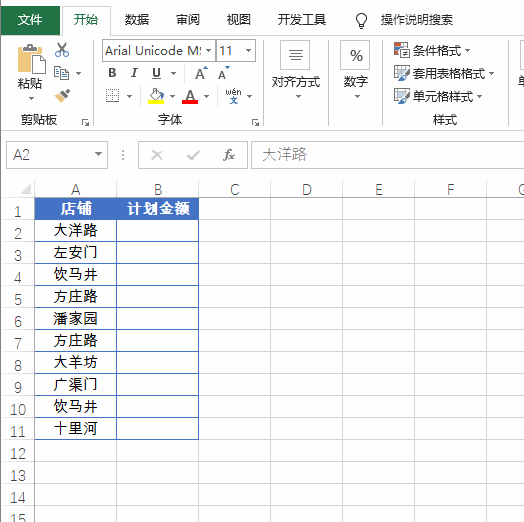# 九个公式很简单，月薪起步两千三

## 1. 条件查询

=VLOOKUP(A2,薪资标准!A:C,3,0)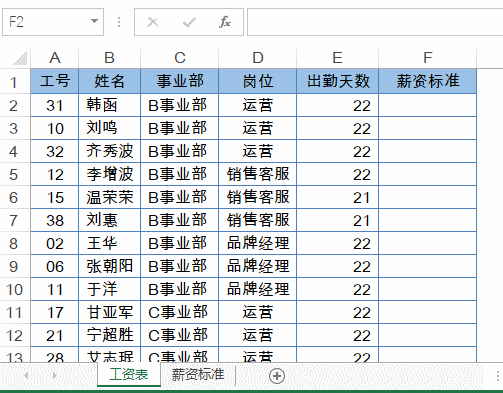VLOOKUP以A2单元格中的工号作为查找值，在薪资标准表中查询出对应的记录。

## 2. 条件计数

=COUNTIF(E:E,”>8000″)/COUNT(E:E)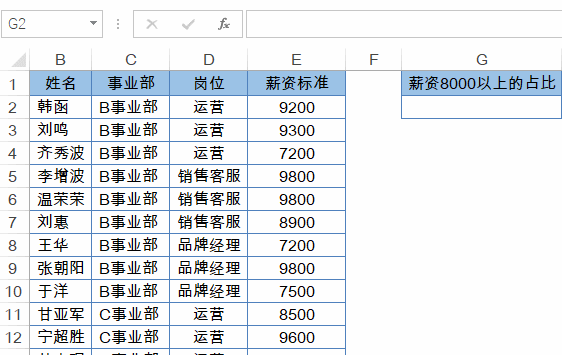## 3. 条件求和

=SUMIF(B:B,F2,D:D)## 4. 有道翻译

=FILTERXML(WEBSERVICE(“http://fanyi.youdao.com/translate?&i=”&A2&”&doctype=xml&version”),”//translation”)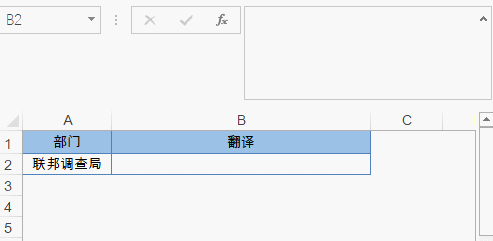## 5. 合并单元格计数

=COUNTA(B2:B13)-SUM(D3:D13)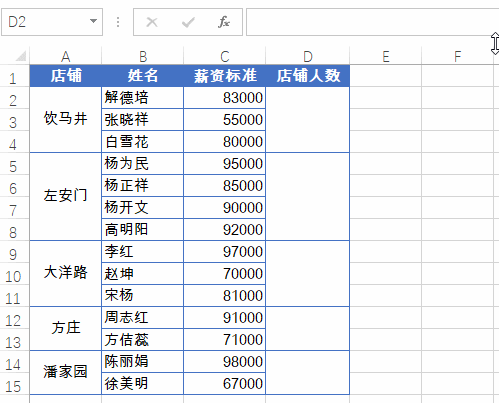## 6. 合并单元格添加序号

=COUNTA(B\$2:B2)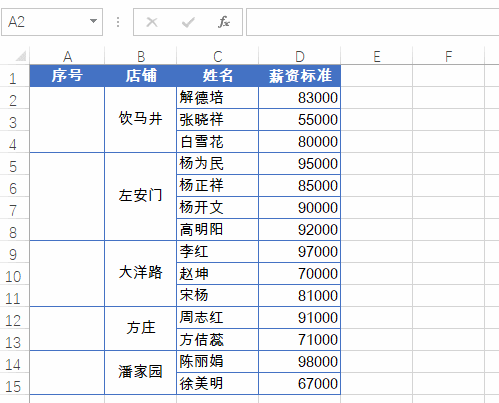## 7. 合并单元格求和

=SUM(C2:C20)-SUM(D3:D20)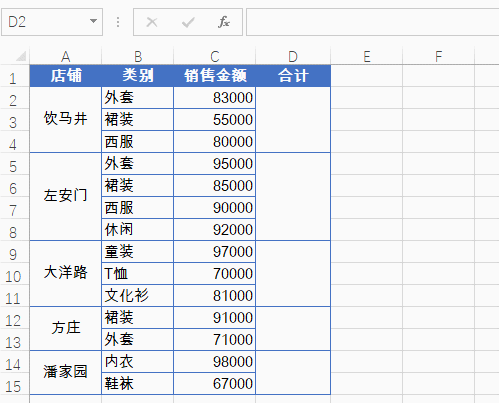SUM(C2:C20)即当前行及以下所有C列数据的和，SUM(D3:D20)是本类别之后所有类别之和，二者相减，得到是本类别的和。

## 8. 限制录入重复数

1、选中要输入数据的A2:A10单元格区域，【数据】→【数据验证】

2、允许类型选择“自定义”，公式为：

=COUNTIF(A:A,A2)=1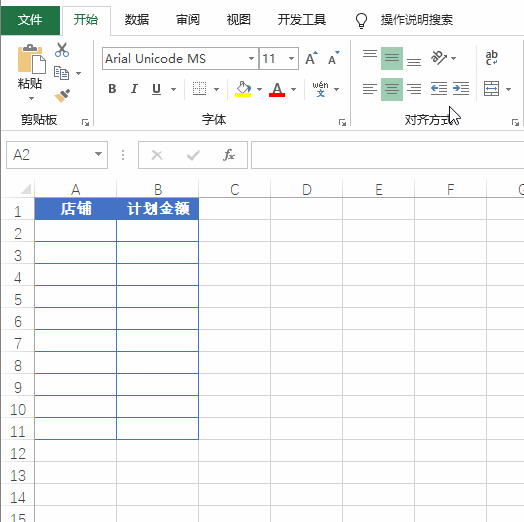## 9. 突出显示重复数据

1、选中已录入数据的A2:A10单元格区域，新建格式规则

2、使用公式：

=COUNTIF(A\$2:A2,A2)>1

3、设置突出显示的格式